# cantilever with uniform distributed load

In this tutorial we will:

• investigate shearing forces and bending moments for a cantilever beam with uniformly distributed load w
• establish general relationships between V, M and w

Consider this example of a cantilever beam 8 m long with uniformly distributed load w kN/m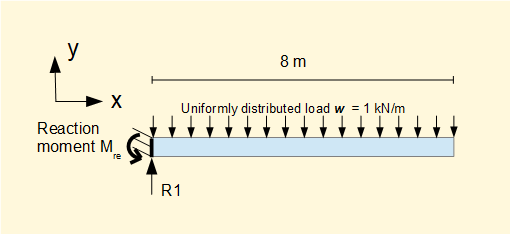A uniformly distributed load is equivalent to a point load at the load centre.  In this case the load centre is the centroid of a rectangle in the x-y plane.

The equivalent point load is (8 m) x (1 kN/m) = 8 kN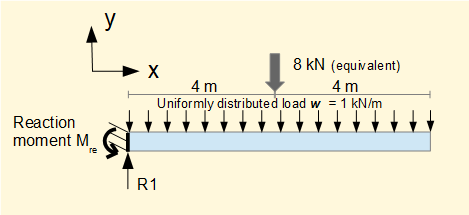Reaction force R1 and reaction moment Mre are calculated by Σ forces = 0 and Σ moments = 0

Σ forces = 0    (upward forces +ve)

R1 - 8 = 0        Thus     R1 = 8 kN

Σ moments = 0    (take moments about the fixed support at the left hand end - clockwise moments +ve)

(8 x 4) ± Mre = 0    Thus     M = -32 kNm (anti-clockwise moment)

We will now calculate shearing forces V and bending moments M along the beam using free body diagrams from datum x = 0.

Firstly consider points x = 0 and x = 8

at x = 0 there is a step change from V = 0 to V = ±8 kN.  The mode of shear at the fixed support is +ve thus V = 8 kN.     The absolute value of the bending moment M at the fixed support is 32 kNm (equal to reaction moment Mre ).  The mode of bending is negative thus M = -32 kNm.

at x = 8 (the right hand end of the beam)  shear force and bending moment cannot be sustained thus   V = 0    and     M = 0

Now consider, say, free body diagrams for sections of the beam from x = 0 to  x = 2, x = 4, and x = 6 and take Σ forces = 0 and Σ moments = 0 at these points.

Assume that we do not know the signs of V and M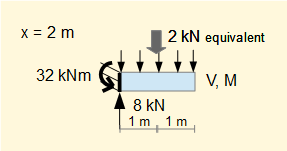Σ forces = 0

8 -2 + V = 0    thus V (appears to) =  -6 kNm  but change sign according to convention.

Thus V = 6 kN

Σ moments = 0

(8 x 2)  - (2 x 1) -32 + M = 0    thus M (appears to) = +18 kNm but change sign according to convention.

Thus M = -18 kNm

The free body diagram is as follows showing negative bending and positive shear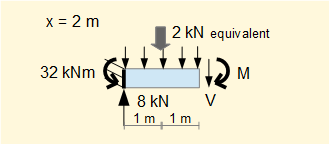Sections at x = 4 and x = 6 follow below.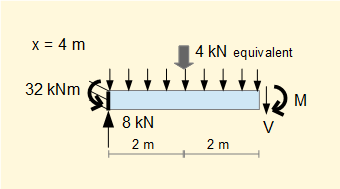V = 4 kN    M = -8 kNm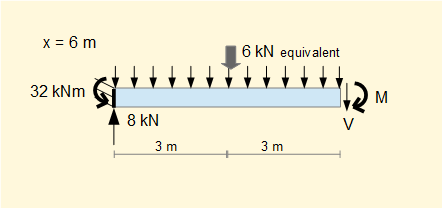V = 2 kN        M = -2 kNm

The above values for V and M plus calculated values for   x = 1    x = 3    x = 5    x = 7  are tabulated below.

 x (m) V (kN) M (kNm) 0 8 -32 1 7 -24.5 2 6 -18 3 5 -12.5 4 4 -8 5 3 -4.5 6 2 -2 7 1 -0.5 8 0 0

Before constructing the shearing force diagram consider changes (ΔV) in V along the beam recalling that the distributed load is w kN/m.

 Section of beam ( Δx = 1 m) ΔV (kN) x = 0 to 1 -1 x = 1 to 2 -1 x = 2 to 3 -1 x = 3 to 4 -1 x = 4 to 5 -1 x = 5 to 6 -1 x = 6 to 7 -1 x = 7 to 8 -1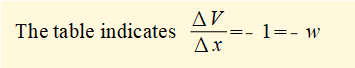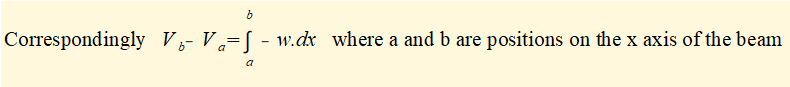Below is the shearing force diagram with slope V(x) =  -1 = --w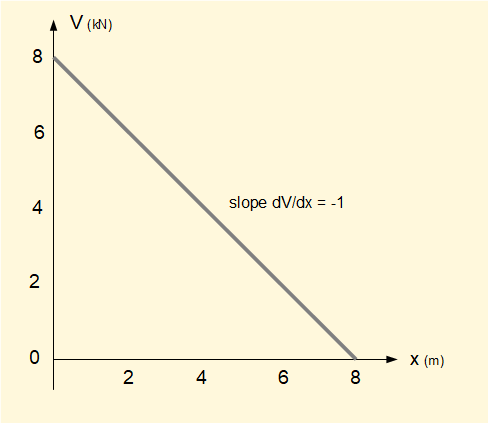Before constructing the bending moment diagram consider changes ( ΔM) in M along the beam

 Section of beam ( Δx = 1 m) ΔM x = 0 to 1 7.5 x = 1 to 2 6.5 x = 2 to 3 5.5 x = 3 to 4 4.5 x = 4 to 5 3.5 x = 5 to 6 2.5 x = 6 to 7 1.5 x = 7 to 8 0.5

Note that the change in ΔM between each interval Δx is -1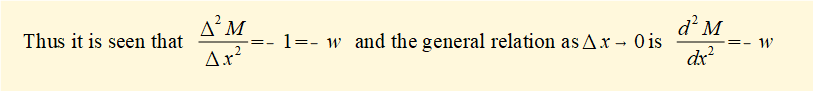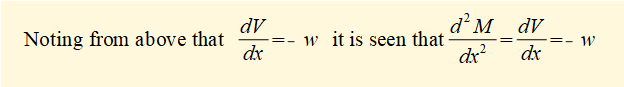Using the calculated values of M at specific points along the beam we can construct an approximate bending moment diagram as follows.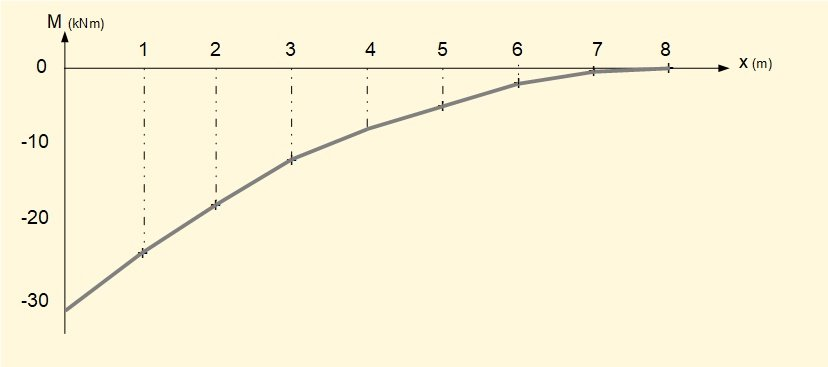The diagram is approximate because we have linked the calculated values of M at specific points with straight lines of slope ΔM/Δx whereas M is really a quadratic function of x. We will now find this function using the general relationship .d2M/dx2 = -w.

We use the general model below for a beam length L with uniformly distributed load w.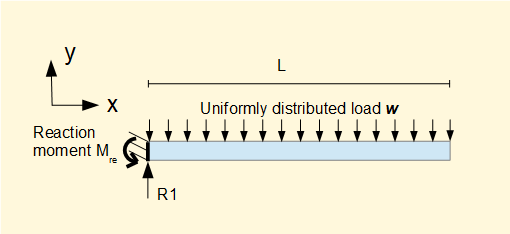From previous work above we know that the load centre of the distributed load occurs at x = L/2.

Thus the reaction moment (at point x = 0) Mre = -wL2/2

The corresponding bending moment M at x = 0 is -wL2/2  (sign convention for negative bending)

At M = L we  know that M = 0 and dm/dx = 0

Now use the general relation d2M/dx2 = -w and then integrate twice to find M as a function of x.  These are general integrals (no defined limits) so we must include constants of integration.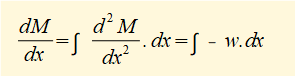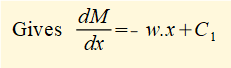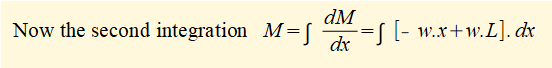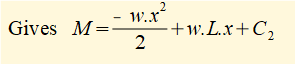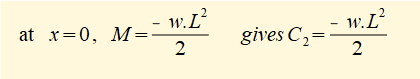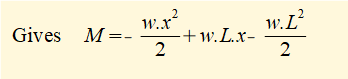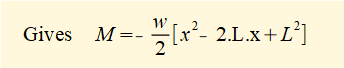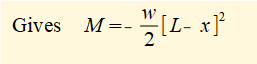For the example used in this tutorial L = 8 and w = 1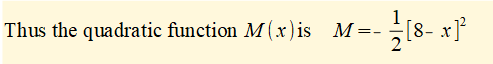The screenshot below plots this function.  The part of the plot which applies is 0 ≤ x ≤ 8 and -32 ≤ M ≤ 0.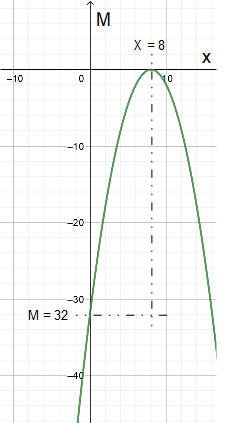I welcome feedback at:

### Tutorials - mechanical vibrations

###### Forced vibrations with damping

Alistair's tutorials 2019